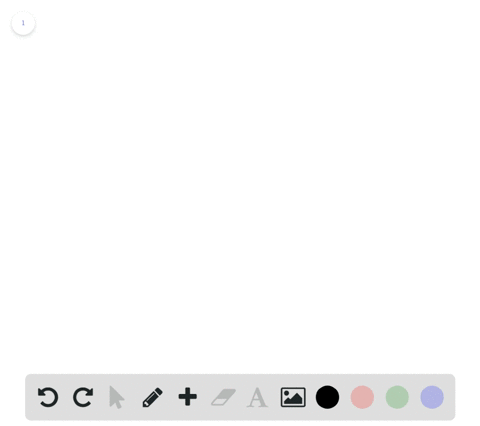🎉 The Study-to-Win Winning Ticket number has been announced! Go to your Tickets dashboard to see if you won! 🎉View Winning Ticket## Discussion

You must be signed in to discuss.

## Video Transcript

problem 16 asks us to evaluate. The six triggered a metric functions of Fada for part A and for part B. And one thing we can take advantage of when looking at this problem is the properties of CO terminal angles. SOCO terminal angles are angles that start and end of the same spot. So although this is ah to single right, it's co terminal angle is actually gonna be this acute angle right here. Same goes for this one. And this is nice for these questions because it might be a little daunting to think about our problem when looking at this huge of two single. But instead, we can just draw a nice triangle for this co terminal angle and then use those values to solve for our chicken metric functions. Sober party. Let's draw a right triangle for this line right here. Is that horizontal on the X axis and this is our theta Keiko terminal. With this Ray. Now we know that in the ex direction there's eight spaces. Okay, goes eight and then in the wind direction and drops down 15. Yeah, and then we can d'oh the Pythagorean theorem to figure out what this Ah, side over here. The site like this. So you would do eight squared Those 15 square equal C squared or C equals the square root of eight squared plus 15 squared. So take a second to figure out what that is. All right, so that's gonna be 17. So with all of our sides figured out, we can easily come over here and then right out with these. Ah, with these triggered a metric equations, they're going to evaluate too. So sign is the same as the opposite side over the high pot news. So 15 over 17 co sign is the adjacent side over the abundance. That ratio is equal to cosign data. So have eight over 17. I pointed towards 15 but I meant 17 tangent is opposite over a Jason. So have 15 over eight now for our next three. They're just the reciprocal sze of these set up here. So co seeking is the reciprocal of sign, meaning that it's 17 over 15. Seeking is the reciprocal of co sign immunity. It's 17 over eight and co tension is the reciprocal of tangent, meaning it is eight over 15. All right, so that those are the six string metric functions for part A. So for a little a up here now moving on to be we're gonna do the exact same process. Okay, so let's create our right triangle with this being angle singles. Arteta. Now we know this side length is one. And this I like this one because it comes out one of the extraction and one in the wind direction. And that means that our hypotheses value when we do, our Pythagorean theorem of a squared plus B squared equals C squared. She's gonna be one squared too close to the top. One squared plus one square equals C squared. Right? See, being this high partners right here, we're gonna end up having C equals the square root of two, this one plus one. Now that we have all of our side links, you can come in and do the same thing we did over here. We can evaluate thes by looking at their ratios. So we have one over square root too. Coastlines going to be one over square to a swell tangent is going to be one over one, which equals one now. Coast seeking will be the reciprocal of sign seeking only the reciprocal of co sign and Coach Angel be their simple of one, which is also just one. All right. And there you have it. Those are the six geriatric functions for both values of theta.